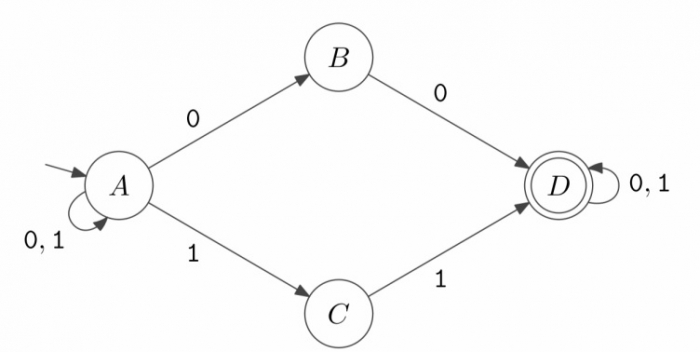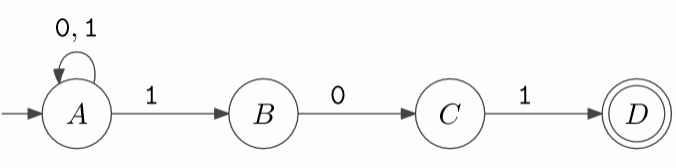# What is Non deterministic finite automata?

For each state, there is exactly one transition corresponding to each symbol of the respective alphabet. This is termed as the Deterministic Finite Automaton (DFA)

## Nondeterministic Finite Automaton (NFA)

For each state there can be zero, one, two, or more transitions corresponding to a particular symbol.

If NFA gets to a state with more than one possible transition corresponding to the input symbol, we say it branches.

If NFA gets to a state where there is no valid transition, then that branch dies An NFA accepts the input string if there exists some choice of transitions that leads to ending in an accept state.

Thus, one accepting branch is enough for the overall NFA to accept, but every branch must reject for the overall NFA to reject.

This is a model of computation. We write DFA to specify a deterministic finite automaton Formally, NFA is 5-tuple (Q, ∑, q0 , T, δ) whereas before

• Q is finite set of states
• ∑ is alphabet of input symbols
• q0 is start state
• T is subset of Q giving the accept states
• δ is the transition function.
• Now the transition function specifies a set of states rather than a state: it maps Q☓ ∑ to {subsets of Q}

## Example 1

NFA accepts any binary string that contains 00 or 11 as a substring. This is shown below −## Example 2

NFA accepts all binary strings that end with 101. This is shown below −